# Expected value facts for kids

Kids Encyclopedia Facts

In probability theory and statistics, the expected value of a random variable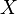$X$ (represented by the symbol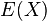$E(X)$) is the average value the variable will take, that is, assuming that the experiment is repeated an infinite number of times, and the mean (or weighted average) of all the values is calculated along the way.

By definition, the expected value of a discrete random variable$X$ is calculated by the formula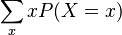$\sum_x x P(X=x)$, where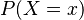$P(X=x)$ is the probability that$X$ equals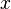$x$, and$x$ ranges over all possible values of$X$.

The Law of large numbers describes how this happens.

## Related pagesExpected value Facts for Kids. Kiddle Encyclopedia.